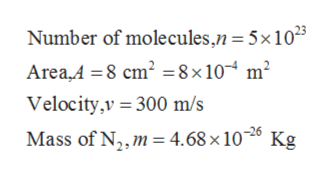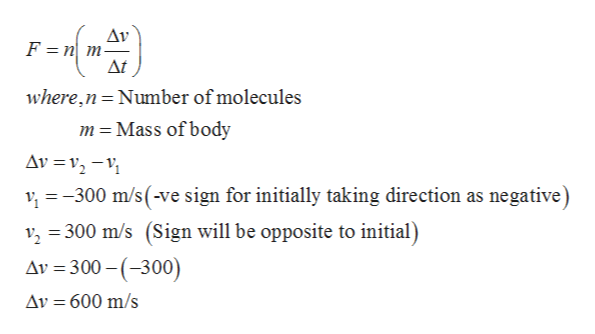# In a period of 1.0 s, 5.0 x 1023 nitrogen molecules strike a wall of area 8.0 cm2. If the molecules move at 3.00 x 102 m/s and strike the wall head-on in a perfectly elastic collision, find the pressure exerted on the wall. (The mass of one N2 molecule is 4.68 x 10-26 kg.)

Question
7 views

In a period of 1.0 s, 5.0 x 1023 nitrogen molecules strike a wall of area 8.0 cm2. If the molecules move at 3.00 x 102 m/s and strike the wall head-on in a perfectly elastic collision, find the pressure exerted on the wall. (The mass of one N2 molecule is 4.68 x 10-26 kg.)

check_circle

Step 1

Given,help_outlineImage TranscriptioncloseNumber of molecules,n=5×10²³ Area,A =8 cm² =8×10 m² Velocity,v = 300 m/s Mass of N,, m = 4.68 × 106 Kg fullscreen
Step 2

Since the collision of molecule is perfectly elastic collision so velocity will be remain same even after collision but direction will change. Force can be calculated using Newton’s Second law,help_outlineImage TranscriptioncloseAv F = n m- At where,n=Number of molecules m = Mass of body Av =v, –V; v, = -300 m/s(-ve sign for initially taking direction as negative) v, = 300 m/s (Sign will be opposite to initial) Av = 300 – (-300) %3D Av = 600 m/s fullscreen
Step 3

By plugging in the given values to...

### Want to see the full answer?

See Solution

#### Want to see this answer and more?

Solutions are written by subject experts who are available 24/7. Questions are typically answered within 1 hour.*

See Solution
*Response times may vary by subject and question.
Tagged in

### Physics